# HC Verma Solutions Class 11 Chapter 17 Light Waves

HC Verma Solutions Class 11 Chapter 17 Light waves are the best source of answers to all the problems discussed in the HC Verma book. The solutions offer insights into various topics of light waves such as questions related to wavelength, frequency and speed of light etc. This chapter also contains some important topics from which questions are framed in the competitive examinations such as IIT JEE. Some of the important problems given in this chapter include:

• Questions on the velocity of light in vacuum and another medium.
• Finding the fringe width and distance of the centre of minimum from the centre of maximum and number of fringes.
• Problems on finding the angular separation between bright fringes.
• Concepts of optical path difference are covered and questions on Young’s double slit experiment are present.
• Students will also find questions on finding the wavelength when intensity is provided along with questions on constructive interference and amplitude of the resulting wave.

While there are many more questions to deal with we are offering free solutions to help students understand the concepts clearly and develop better problem-solving skills. These solutions will further help students understand the chapter in a better way and have a fun learning experience.

Get to know more about topics like;

1. Waves or Particles
2. The Nature of Light Waves
3. Hiiygens’ Principle
4. Young’s Double Hole Experiment
5. Young’s Double Slit Experiment
6. Optical Path
7. Interference from Thin Films
8. Fresnel’s Biprism
9. Coherent and Incoherent Sources
10. Diffraction of Light
11. Fraunhofer Diffraction by a Single Slit
12. Fraunhofer Diffraction by a Circular Aperture
13. Fresnel Diffraction at a Straight Edge
14. Limit of Resolution
15. Scattering of Light
16. Polarization of Light

## Class 11 Important Questions In Chapter 17

1. Is the interference phenomenon a proof of light being a longitudinal wave or a transverse wave? Justify your answer.

2. White light is beamed on a double slit. The condition here is that one slit is covered by a violet filter which allows X = 400 nm. Describe your observation and the nature of the fringe pattern that is formed.

3. When there is a refraction of light which factor is not affected or what does not change?

(a) Velocity (b) Amplitude (c) Wavelength (d) Frequency

4. The best monochromatic light can be achieved from which source?

(a) Laser (b) Mercury Tube (c) Bulb (d) Candle

5. What will happen when a fringe-width when a thin transparent sheet is placed in front of Young’s double slit.

(a) decrease (b) increase (c) remain the same (d) become non-uniform.

## HC Verma Solutions Vol 1 Light Waves Chapter 17

Question 1: Find the range of frequency of light that is visible to an average human being (400 nm < λ < 700 nm).

Solution:

We know, c = vλ

Where c = speed of light = 3 x 108 m/s2

Minimum wavelength = λmin = 400 nm

Associated frequency = vmax = [3 x 108]/[400 x10-9] = 7.5 x 1014 Hz

Max wavelength = λmax = 700 nm

and vmin = [3 x 108]/[700 x10-9] = 4.3 x 1014 Hz

Range of the frequency = 4.3 x 1014 Hz to 7.5 x 1014 Hz

Question 2: The wavelength of sodium light in air is 589 nm. (a) Find its frequency in air. (b) Find its wavelength in water (refractive index = 1.33). (c) Find its frequency in water. (d) Find its speed in water.

Solution:

(a) frequency = vNa = c/λNa = [3×108]/[589×109] = 5.09×1014 Hz

(b) Wavelength of sodium light in water = λNa’ = λNa/μ = 589/1.33 = 443 nm (approx)

(c)Frequency of light does not change, i.e. 5.09×1014 Hz

(d) speed of light = c’ = c/μ = [3×108]/1.33 = 2.25×108 m/s

Question 3: The index of refraction of fused quartz is 1.472 for light of wavelength 400 nm and is 1.452 for light of wavelength 760 nm. Find the speeds of light of these wavelengths in fused quartz.

Solution:

The speed of light in quartz = c’ = c/μ = [3×108]/1.472 = 2.04 x 108 m/s

Here, wavelength = 400nm

The speed of light in quartz = [3×108]/1.452 = 2.07 x 108 m/s

Here, wavelength = 760nm

Question 4: The speed of the yellow light in a certain liquid is 2.4 × 108 m s-1. Find the refractive index of the liquid.

Solution:

Speed of light in a medium of refractive index “μ ”

Here c’ = 2.04 x 108 m/s

We know, c = speed of light = 3 x 108 m/s2

Now, c’ = c/μ

or μ = c/c’ = [3×108]/[2.4×108] = 1.25

Question 5: Two narrow slits emitting light in phase are separated by a distance of 1.0 cm. The wavelength of the light is 5.O × 10-7 m. The interference pattern is observed on a screen placed at u distance of 1.0 m. (a) Find the separation between the consecutive maxima. Can you expect to distinguish between these maxima? (b) Find the separation between the sources which will give a separation of 1.0 mm between the consecutive maxima.

Solution:

Distance between the slits = d = 1 cm

Distance between the slits and the screen= D = 1 m

Wavelength of the light = λ = 5×10-7 m

(a)

w = Dλ/d

w = [1x5x10-7]/0.01 = 0.05 mm

(b) Here w = 1 mm

So, d = Dλ/w = [1x5x107]/0.001 = 0.5 mm

Question 6: The separation between the consecutive dark fringes in a Young’s double slit experiment is 1.0 mm The screen is placed at a distance of 2.5 m from the slits and the separation between the slits is 1.0 mm. Calculate the wavelength of light used for the experiment.

Solution:

The width of a fringe = w = 1mm = 0.001 m

Distance between the screen and the slit = D = 2.5 m

Separation between the slits = d = 10mm = 0.01 m

We know, w = Dλ/d

=> λ = dw/D = [0.001 x 0.01]/2.5 = 400 nm

Question 7: In a double slit interference experiment, the separation between the slits is 1.0 mm, the wavelength of light used is 5.0 × 10-7 m and the distance of the screen from the slits is 1.0 m. (a) Find the distance of the center of the first minimum from the center of the central maximum. (b) How many bright fringes are formed in one centimetre width on the screen?

Solution:

Distance between the screen and the slit = D = 1 m

Separation between the slits = d = 1mm = 0.001 m

wavelength of light = 5×10-7 m

(a)

We know, w = Dλ/2d

=[1x5x10-7]/[2×0.001] = 0.25 mm

(b) width of one fringe = w = 0.5 mm

Number of such fringes present in 1 cm region = 10/0.5 = 20

Question 8: In a Young’s double slit experiment, two narrow vertical slits placed 0.800 mm apart are illuminated by the same source of yellow light of wavelength 589 nm. How far are the adjacent bright bands in the interference pattern observed on a screen 2.00 m away?

Solution:

Distance between the screen and the slit = D = 200 m

Separation between the slits = d = 0.8 mm = 0.0008 m

wavelength of light = 589×10-9 m

We know, w = Dλ/d

= [200 x 589×10-9]/0.0008 = 1.47 mm (approx)

Question 9: Find the angular separation between the consecutive bright fringes in a Young’s double slit experiment with blue-green light of wavelength 500 nm. The separation between the slits is 2.0 × 10-3 m.

Solution:

Separation between the slits = d = 2.0 × 10-3 m

wavelength of light = 500×10-9 m

We know, d sinθ = λ

For small angle, sinθ = θ

Now, θ = [500 x10-9]/[2×10-3] rad = 0.0014 degree (approx.)

Question 10: A source emitting light of wavelengths 480 am and 600 nm is used in a double slit interference experiment. The separation between the slits is 0.25 mm and the interference is observed on n screen placed at 150 cm from the slits. Find the linear separation between the first maximum next to the central maximum) corresponding to the two wavelengths.

Solution:

Separation between the slits = d = 0.25 mm = 0.25 × 10-3 m

Distance between the screen and the slit = D = 150 cm = 1.5 m

wavelength of light = 500×10-9 m

We know, w = Dλ/d

For first time occur, wavelength is 480 x10^-9 m

w1 = Dλ/d = [1.5x480x10-9]/[0.2×10-3]

For wavelength = 600 x 10-9 m

w2 = [1.5 x 600 x10-9]/[0.2×10-3]

The separation between these two bright fringes is = w2 – w1 = 0.72 mm

Question 11: White light is used in a Young’s double slit experiment. Find the minimum order of the violet fringe (λ = 400 nm) which overlaps with a red fringe (λ = 700 nm).

Solution:

The distance of nth fringe from the centre = w = nDλ/d

Let mth violet fringe overlaps with the nth red fringe, then

nDλv/d = nDλr/d

=> m/n = λrv = 700/400 = 7/4

Question 12: Find the thickness of a plate which will produce a change in optical path equal to half the wavelength λ of the light passing through it normally. The refractive index of the plate is μ.

Solution:

Let Δx be the thickness of the plate.

μ Δx – Δx = λ/2

=> Δx = λ/[2(μ-1)

Question 13: A plate of thickness t made of a material of refractive index μ is placed in front of one of the slits in a double slit experiment. (a) Find the change iii the optical path due to introduction of the plate. (b) What should be the minimum thickness t which will make the intensity at the center of the fringe pattern aero? Wavelength of the light used is λ. Neglect any absorption of light in the plate.

Solution:

(a) The optical path length in vacuum is “t” and that introduce due to the plate is “μt” .

change in optical path length = μt – t

(b) To have a dark fringe at the centre the pattern should shift by one half of a fringe.

μt – t = λ/2

=> t = λ/[2(μ-1]

Question 14: A transparent paper (refractive index = 1.45) of thickness 0.02 mm is pasted on one of the slits of a Young’s double slit experiment which uses monochromatic light of wavelength 620 nm. How many fringes will cross through the center if the paper is removed?

Solution:

Optical path difference = μt – t

If there was no film present, there is no path difference at the center. But due to the presence of the film, we have a path difference of λ.

For path difference λ we have 1 fringe shift.

For path difference μt – t we have (μt – t)/λ fringe shift.

=> μt – t = nλ

=> n = [μt-t]/λ = [(1.45-1)0.02×10-3]/[620×10-9] = 14.5 fringes (approx)

Question 15: In a Young’s double slit experiment using mono-chromatic light, the fringe pattern shifts by a certain distance on the screen when a mica sheet of refractive index 1.6 and thickness 1.964 micron (1 micron = 10-6 m) is introduced in the path of one of the Interfering waves. The mica sheet is then removed and the distance between the screen and the slits is doubled. It is found that the distance between the successive maxima now is the same as the observed fringe-shift upon the introduction of the mica sheet. Calculate the wavelength of the monochromatic light used in the experiment.

Solution:

The number of fringe shifted = n = (μ-1)t/λ

Corresponding shift = Number of fringes shifted x fringe width

= (μ-1)t/λ x λD/d

= (μ-1)tD/d

When the distance between the screen and the slits is doubled

Fringe width = λ(2D)/d

Form above equations,

(μ-1)tD/d = λ(2D)/d

=> λ = [(1.6-1)x(1.964×10-6)]/2 = 589.2 nm

Question 16: A mica strip and a polystyrene strip are fitted on the two slits of a double slit apparatus. The thickness of the strips is 0.50 mm and the separation between the slits is 0.12 cm. The refractive index of mica and polystyrene are 1.58 and 1.55 respectively for the light of wavelength 590 nm which is used in the experiment. The interference is observed on a screen a distance one meter away. (a) What would be the fringe-width? (b) At what distance from the center will the first maximum be located?

Solution:

(a)The fringe width = w = Dλ/d

=> w = [1x590x10-9]/[0.12×10-2]

= 4.9 x 10-4 m

(b) The optical path difference:

Δx = μm t – μp t

μm = refractive index of mica and μp = refractive index of polystyrene

Δx = (1.58 – 1.55)5 x 10-4

= 1.5 x 10-5 m

We know, Δx = nλ

Number of fringe shifts = n = Δx/λ

= [1.5×10-5]/[590×10-9]

= 25.42

There are 25 fringes and 0.42th of a fringe.

Therefore,

maximum on one side = 0.42w = 0.021 cm

another side = 0.58w = 0.028 cm

[using value of w]

Question 17: Two transparent slabs having equal thickness but different refractive indices μ1 and μ2 are pasted side by side to form a composite slab. This slab is placed just after the double slit in a Young’s experiment so that the light from one slit goes through one material and the light from the other slit goes through the other material. What should be the minimum thickness of the slab so that there is a minimum at the point P0 which is equidistant from the slits?

Solution:

The change of path difference due to the two slabs = (μ1 – μ2)t

For having a minimum at Po, the path difference should change by λ/2

=> λ/2 = (μ1 – μ2)t

=> t = λ/[2(μ1 – μ2)]

Question 18: A thin paper of thickness 0.02 mm having a refractive index 1.45 is pasted across one of the slits in a Young’s double slit experiment. The paper transmits 4/9 of the light energy falling on it.

(a) Find the ratio of the maximum intensity to the minimum intensity in the fringe pattern.

(b) How many fringes will cross through the center if an identical paper piece is pasted on the other slit also? The wavelength of the light used is 600 nm.

Solution:

I = original intensity of light and l’ = intensity after passing from the paper.

l’ = (4/9)l

=> l’/l = 4/9

Again, we know

l’/l = a’2/a2

Where a be the initial amplitude and that from the paper be a’.

=>

l’/l = a’2/a2 = 4/9

a’/a = 2/3 = k (constant)

Therefore, a’ = 2k and a = 3k

For maximum amplitude = a’ + a = 5k

For minimum amplitude = a’ – a = k

Ratio of maximum intensity to minimum:

lmax/lmin = (5k) 2/k2 = 25

(b) μ (refractive index) = 1.45

Wavelength of light(λ) = 600×10-9 m

t = 0.02×10-2

optical path difference = (μ-1)t = nλ

using values, we get

=> n = 15

Question 19: A Young’s double slit apparatus has slits separated by 0.28 mm and a screen 48 cm away from the slits. The whole apparatus is immersed in water and the slits are illuminated by the red light (λ = 700 nm in vacuum). Find the fringe-width of the pattern formed on the screen.

Solution:

Distance between the slits = d = 0.28 mm = 0.28 x 10-3 m

Distance between the slits and the screen= D = 48 cm = 0.48 m

Wavelength of the light = λ = 700×10-9 m

Refractive index of water= μ = 1.33

We know, fringe width = w = Dλ/d

As light is passing through water, its wavelength changes. So, the new wavelength is

λ’ = λ/μ = [700×10-9]/1.33 = 5.26×10-7 m

Hence,

w = Dλ’/d = [0.48×5.26×10-7]/[0.28 x 10-3]

= 0.9 mm

Question 20: A parallel beam of monochromatic light is used in a Young’s double slit experiment. The slits are separated by a distance d and the screen is placed parallel to the plane of the slits. Show that if the incident beam makes an angle

θ = sin-1(λ/2d) with the normal to the plane of the slits, there will be a dark fringe at the center Po of the pattern.

Solution:

From figure, the wavefronts are making an angle with the normal to the slit passing through S1 and S2.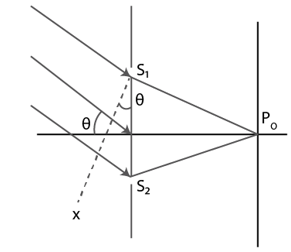In right triangle M S1 S2, at M

sinθ = MS1/S1S2

=> MS1 = S1S2 sin θ = d sin θ

If the path difference is λ/2

Then d sin θ= λ/2

=> θ = sin-1(λ/2d)

Question 21: A narrow slit S transmitting light of wavelength λ is placed a distance d above a large plane mirror as shown in figure (below). The light coming directly from the slit and that coming after the reflection interfere at a screen ∑ placed at a distance D from the slit. (a) What will be the intensity at a point just above the mirror, i.e., just above O? (b) At what distance from O does the first maximum occur?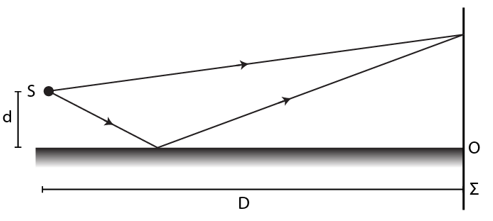Solution: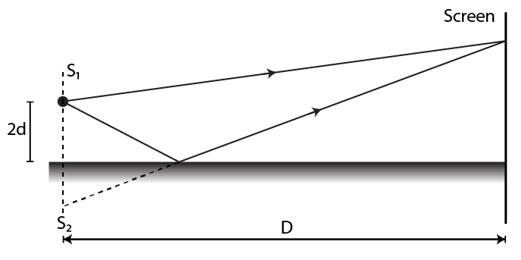(a) There is a phase difference of π between direct light and reflecting light, the intensity just above the mirror will be zero.

(b) 2d = equivalent slot separation and D is the distance between slit and screen.

For bright fringe = Δx = y(2d)/D = nλ

As there is phase reverse of λ/2

=> y(2d)/D + λ/2 = nλ

=> y = λD/4d

Question 22: A long narrow horizontal slit is placed 1 mm above a horizontal plane mirror. The interference between the light coming directly from the slit and that after reflection is seen on a screen 1.0 m away from the slit. Find the fringe-width if the light used has a wavelength of 700 nm.

Solution:

separation between the slit = 2d = 2mm = 22 x 10-3 m

Now, fringe width = w = Dλ/d

=> w = [1x700x10-9]/[2×10-3] = 0.35×10-3 m = 0.35 mm

Question 23: Consider the situation of the previous problem. If the mirror reflects only 64% of the light energy falling on it, what will be the ratio of the maximum to the minimum intensity in the interference pattern observed on the screen?

Solution:

l’/l = a’2/a2 = 64/100

a’/a = 4/5 = k

Here, k is some constant.

Therefore, a’ = 4k and a = 5k.

Now,

For maximum amplitude = a’ + a = 9k

For minimum amplitude = a’ – a = k

Ratio of maximum intensity to minimum:

lmax/lmin = (9k) 2/k2 = 81/1

Question 24: A double slit S1 – S2 is illuminated by a coherent light of wavelength λ. The slits are separated by a distance d. A plane mirror is placed in front of the double slit, at a distance D1 from it and a screen ∑ is placed behind the double slit at a distance D2 from it (figure below). The screen ∑ receives only the light reflected by the mirror. Find the fringe-width of the interference pattern on the screen.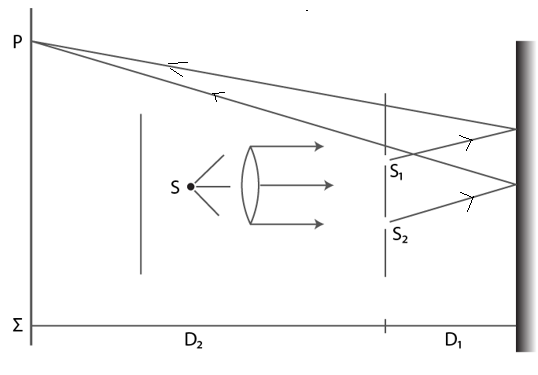Solution:

It’s clear from the figure that, the apparent distance of the screen from the slits is D = 2D1 + D2.

Both rays will be shifted by pi and hence will form a normal interference pattern.

So, fringe width = w = Dλ/d

= [2D1 + D2] λ/d

Question 25: White coherent light (400 nm—700 nm) is sent through the slits of a Young’s double slit experiment (figure below). The separation between the slits is 0.5 mm and the screen is 50 cm away from the slits. There is a hole in the screen at a point 1.0 mm away (along the width of the fringes) from the central line. (a) Which wavelength(s) will be absent in the light coming from the hole? (b) which wavelength(s) will have a strong intensity?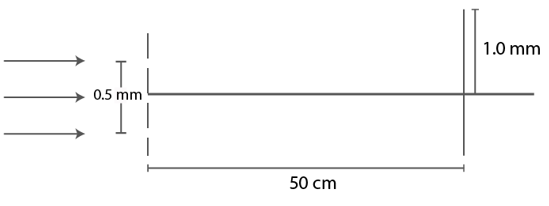Solution:

Distance between the screen and the slit = D = 0.5 m

Separation between the slits = d = 0.5 mm = 0.0005 m

Let Δx be the path difference at a point y above the center on the screen

=> Δx = yd/D …(1)

Also, condition for minima: Δx = (n + 1/2)λ ….(2)

From (1) and (2)

yd/D = (n + 1/2)λ

At y = 1 mm = 0.0001 m

Putting all the values, we have

=> λ = [10-6/(n + 1/2) ] m

For n = 0 => λ = 2000 nm [Out of range]

For n = 1 => λ = 667 nm [in range]

For n = 2 => λ = 400 nm [in range]

For n = 3 => λ = 286 nm [Out of range]

(b) Δx = n λ [for maxima]

Δx = n λ = yd/D

=> λ = yd/Dn

For n = 1 => λ = 1000 nm [in range]

For n = 2 => λ = 500 nm [in range]

For n = 3 => λ = 333 nm [Out of range]

Hence maximum intensity would be for λ = 500 nm

Question 26: Consider the arrangement shown in figure (below). The distance D is large compared to the separation d between the slits. (a) Find the minimum value of d so that there is a dark fringe at O. (b) Suppose d has this value. Find the distance x at which the next bright fringe is formed. (e) Find the fringe-width.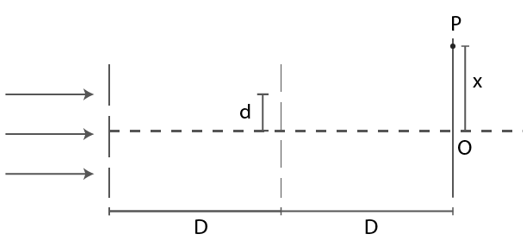Solution: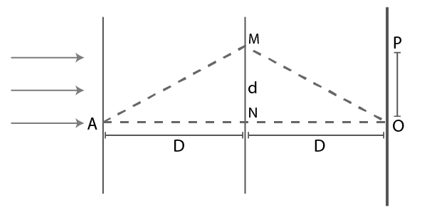Form figure,

Δx = AMO – ANO

Here AM = MO = √[D2 + d2] AND AN = NO = D

=> Δx = 2(AM – AN) = 2 {√[D2 + d2] – D}

For minima at O,

2 {√[D2 + d2] – D} = (n +(1/2)) λ

Solving above equation for d, we get

d = √[Dλ/2]

(b) width of the dark fringe = w = Dλ/d

Now, the location x is given by

x = Dλ/[2√(Dλ/2)]

=> x = d

(c) As x = w/2

=> w = 2x = 2d

Question 27: Two coherent point sources S1 and S2 vibrating in phase emit light of wavelength λ. The separation between the sources is 2λ. Consider a line passing through S2 and perpendicular to the line S1S2. What is the smallest distance from S2 where a minimum of intensity occurs?

Solution: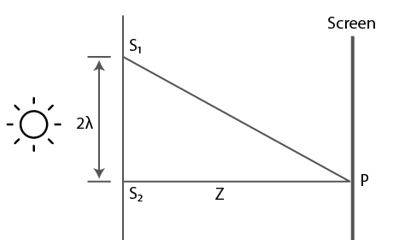For minimum intensity

S1 P – S2 P = x = (2n+1)λ/2

Form diagram,

√[Z2 + (2λ) 2] – Z = (2n+1)λ/2

Taking square both the sides and solving above equation, we have

Z = [16λ2 -(2n+1) 2 λ2]/[4(2n+1)λ]

Now,

For n = -1 => Z= -15λ/4

For n = 0 => Z = 15λ/4

For n = 1 => Z= 7λ/12 and

For n = 2 => Z= -9λ/20

Therefore, Z= 7λ/12 is the smallest distance for which there will be minimum intensity.

Question 28: Figure (below) shows three equidistant slits being illuminated by a monochromatic parallel beam of light. Let BP0 – AP0 = λ/3 and D >> λ. (a) Show that in this case d = √[2λD/3]. (b) Show that the intensity at P0 is three times the intensity due to any of the three slits individually.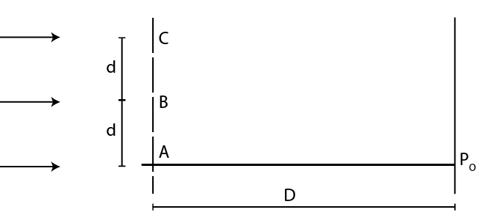Solution:

(a) BP0 – AP0 = λ/3

Form diagram,

BP0 – AP0 = √[D2+d2] – D = λ/3

Solving above equation,

=> d = √[2Dλ/3] [Hint: Ignore λ2/9 from the above expression, as it is small value]

(b) Here d/2 + d = 3d/2, be the distance of P0 from the line in the middle of B and C.

Path difference between waves coming from B and C : 3d/2 x d/D = λ

[Using value of d from part (a)]

Here 2π/3 is the phase difference of the wave coming from A.

If “a” be the amplitude from each slit, then contribution from B and C is 2a.

Therefore, resultant intensity taking in consideration the phase difference:

A2 = (2a) 2 + a2 + 2a2 cos(2π/3) = 5a2 – 2a2 = 3a2

Now, the ratio of total intensity by individual intensity :

ltotal/l = A2/a2 = 3

=> ltotal = 3l . (Hence proved)

Question 29: In a Young’s double slit experiment, the separation between the slits = 2.0 mm, the wavelength of the light = 600 am and the distance of the screen from the slits = 2.0 m. If the intensity at the center of the central maximum is 0.20 W m-2, what will be the intensity at a point 0.5 cm away from this center along the width of the fringes?

Solution:

Distance between the screen and the slit = D = 2 m

Separation between the slits = d = 2 mm = 2 x 10-3 m

Wavelength of light = λ = 600 x 10-9 m

from question, lm/l = 4a2/2

=> l = lm/4

At y = 0.5 cm = 0.005 m, path difference = Δx = yd/D

=> Δx = [0.005 x 0.002]/2 = 5 x 10-6 m

The phase difference:

φ = 2πΔx/λ = 50π/3 = 2π/3

Now, the resultant amplitude, A is:

A2 = a2 + a2 + 2a2 cos(2π/3) = a2

=> A = a

Let the intensity of the resulting wave at point 0.5 cm be I.

=> I/I_max = A2/(2a) 2

=> I/0.2 = 1/4

=> I = 0.2/4 = 0.05 W/m2 .

Question 30: In a Young’s double slit interference experiment the fringe pattern is observed on a screen placed at a distance D from the slits. The slits are separated by a distance d and are illuminated by monochromatic light of wavelength λ. Find the distance from the central point where the intensity falls to (a) half the maximum, (b) one fourth of the maximum.

Solution:

Let Imax = maximum intensity and

I = intensity at y.

(a) Imax/I = 2/1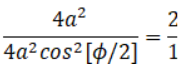cos2[φ/2] = 1/2

=> φ = π/2

The path difference:

Δx = λφ/2π = λ/4

=> y = ΔxD/d = λD/4d

(b) when intensity is (1/4) times the maximum:

Imax/I = 4/1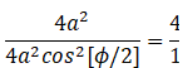cos2 [φ/2] = 1/4

=> φ = 2π/3

The path difference:

Δx = λφ/2π = λ/3

=> y = ΔxD/d = λD/3d